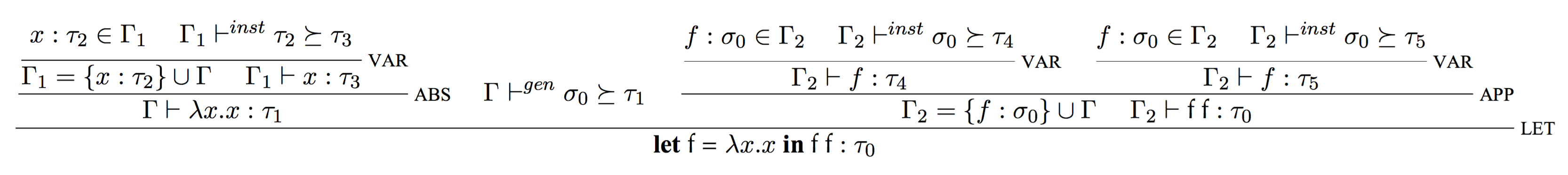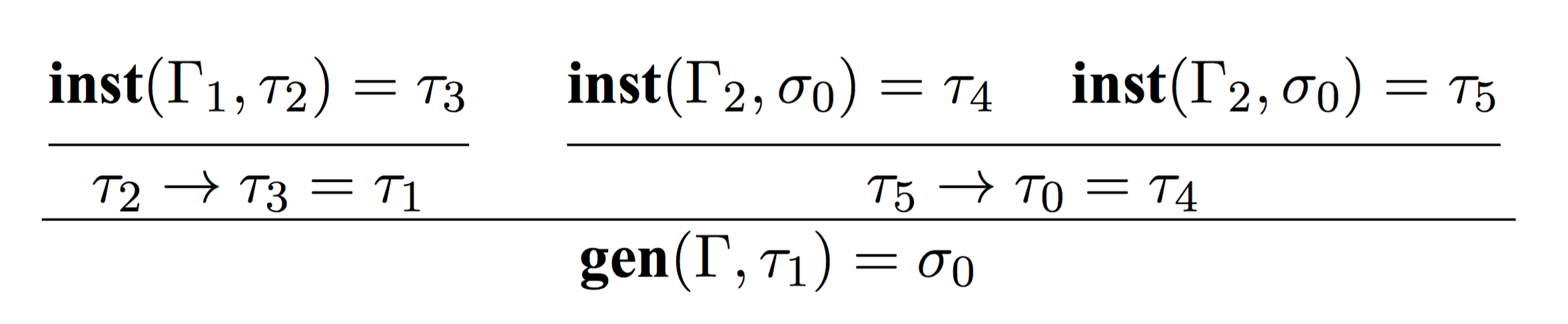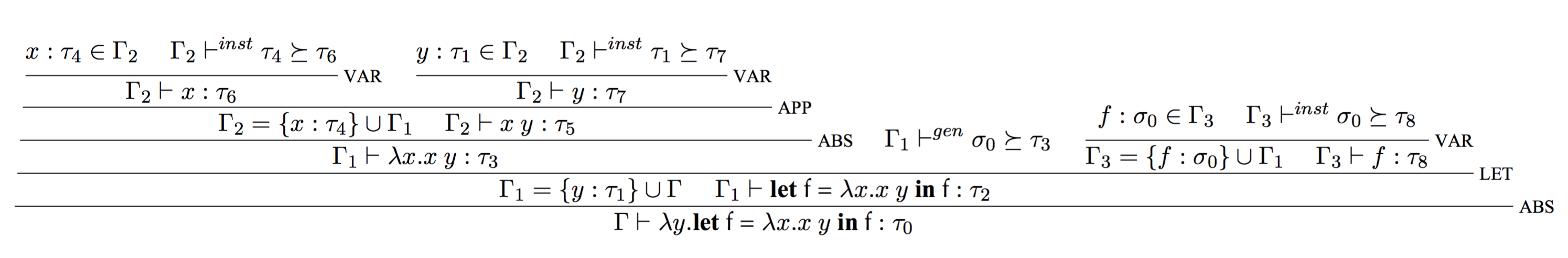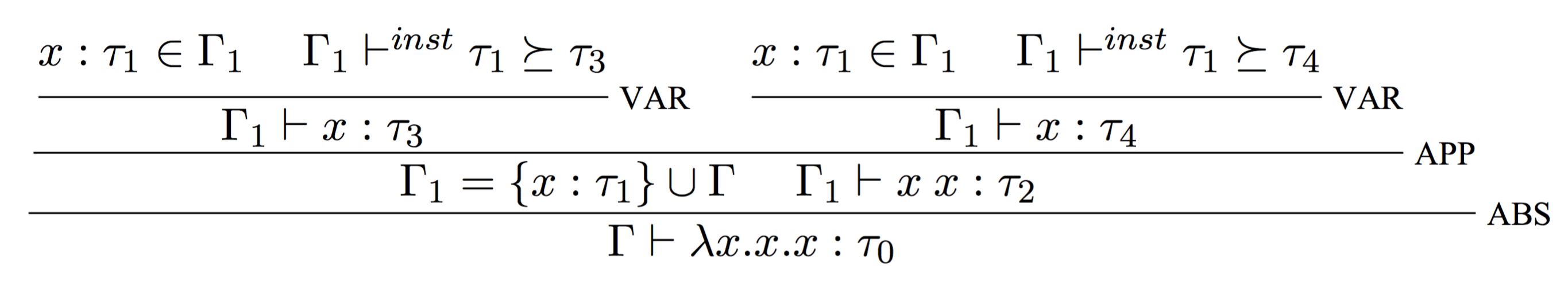@darwin-yuan 2016-07-03T02:49:33.000000Z 字数 10182 阅读 2777

# Hindley-Milner类型系统（3）

类型系统 Haskell

## 语法制导规则

HM类型系统里，产生这两条规则的原因只有一个，那就是let binding。因为只有在let binding里才能做到多态。

$\newcommand{\typerule}{\cfrac{#1}{#2}\scriptstyle{\mathrm{#3}}\displaystyle}$

## 基于约束的推演• 先从 $\textbf{inst}(\Gamma_{1}, \tau_{2}) = \tau_{3}$ 开始，由于$\tau_{2}$不是一个polytype，因而$\tau_{2} = \tau_{3}$
• 然后我们可以从 $\tau_{2} \rightarrow \tau_{3} = \tau_{1}$ 得出 $\tau_{2} \rightarrow \tau_{2} = \tau_{1}$
• 再运用 $\textbf{gen}(\Gamma,\tau_{1}) = \sigma_{0}$，可以得到 $\sigma_{0} = \forall \alpha. \alpha \rightarrow \alpha$
• 有了$\sigma_{0}$，我们就可以从$\textbf{inst}(\Gamma_{2}, \sigma_{0}) = \tau_{4}$推出$\tau_{6} \rightarrow \tau_{6} = \tau_{4}$
• 同样的，从$\textbf{inst}(\Gamma_{2}, \sigma_{0}) = \tau_{4}$推出$\tau_{7} \rightarrow \tau_{7} = \tau_{5}$
• 由于$\tau_{5} \rightarrow \tau_{0} = \tau_{4}$，所以可以推出$\tau_{6} \rightarrow \tau_{6} = \tau_{5} \rightarrow \tau_{0}$；因而：$\tau_0 = \tau_{5} = \tau_{6} = \tau_{7} \rightarrow \tau_{7}$

1. 避免了和环境中已有的自由变量冲突的可能性;
2. 同一个名字f，每次出现，都会产生一次新的实例化，由于实例化后得到的类型是一个monotype。因而f的两次实例化得到的类型是不同的。而这正是$f$实现多态化的关键。

## Unification

$\newcommand{\letexpr}{\textbf{let}\textbf{ #1 = } #2 \textbf{ in }\textbf{#3}:#4}$

## 推演过程中的自由类型变量

$\newcommand{\letexpr}{\textbf{let}\textbf{ #1 = } #2 \textbf{ in }\textbf{#3}:#4}$## 失败的推演

$\lambda$演算理论里，如下等价关系成立：HM类型系统下，一个$\lambda$的参数和结果类型都是monotype，但这与函数自身的类型是否是个polytype没有关系。• 私有
• 公开
• 删除